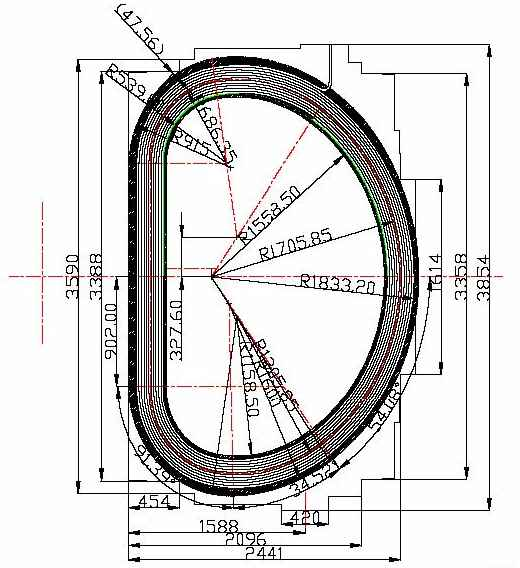Last results > MEMCEI v1.0 Magnetic field and forces using MEMCEI v1.0 January 2005 A finite elements code to calculate magnetic fields and mechanical loads has been developed and it has been named MEMCEI code. Till now it has been tested by recalculating the TF system of the HT-7U Chinese tokamak, however further improvements and comparisons are necessary.     The finite elements code model each current line by a modified Ampere-Laplace law. The exact law is applied outside the theoretical conductor and inside the conductor the law behaves as the magnetic field inside a circular wire loaded with a uniform current. Up to 9600 current element model has been successfully modelled and solved using MEMCEI v1.0. A higher number of elements is being tried in order to increase accuracy but some problems in the code need to be solved.   Magnetic Field    The MEMCEI code has been compared with the TF coils of the HT-7U tokamak, one of the most recent superconductor tokamaks. The TF magnetic field of this tokamak was calculated by the HT-7U team using the EFFI code. The same code was used in Wendelstein 7-X.   Data corresponding to the HT-7U geometrical inputs and results come from   and . The model in HT-7U is divided into 164 current elements, the current is 14,3077 kA to obtain 3.5 T at the plasma center, having 130 turns each TF coil. The torus is composed of 16 TF coils.  In this calculation using MEMCEI v1.0   9600 current elements are calculated, distributed in 16 TF coils and using 12 line current loops per coil that amounts up to 50 current elements each D shape single electrical loop.  The results obtained using the most accurate HT-7U geometry available till now are expressed in the graph 1.   The differences are notable (the exact deviation will be calculate when the exact HT-7U values were available) inside de conductors and near them. This might result from the low number and long finite elements of the model and because the finite elements in the version v1.0 are modeled as spheres.      More accurate knowledge of the modellization of the currents in the EFFI model is necessary to compare the toroidal magnetic field inside the conductors. More elements are necessary and perhaps a new modified Ampere law in order to increase the accuracy near the modelled finite elements, if possible without increasing the number of elements.     Forces   For the standard mode Bt=3.5T , the centering force on each coil is 989.366 ton  , and from MEMCEI is 963.26 ton resulting a 2.5% deviation. When the magnetic field were improved the forces will be correctly calculated.       An interface between the magnetic field calculations and mechanical stress using the French Code_Aster is being developed so to calculate in same kind of evolutionary way the whole system including magnetic, electrical and mechanical specifications.     “Engineering of the HT-7U Superconducting Magnet Systems”, S.T. Wu, et al, Institute of Plasma Physics, Chinese Academy of Sciences.  “The Analysis and Calculation for the Toroidal Magnetic field of HT-7U”, Chen Wenge, et al, Institute of Plasma Physics, C. A. S.  “Design of the HT-7U Tokamak Device”, S.T. Wu, et al, Institute of Plasma Physics, C. A. S.View of the TF coil HT-7U as shown in          Graph 1  :  HT-7U Bt calculations using EFFI and MEMCEI codes.  - Btg is the magnetic field in the toroial axis using EFFI, -Bto(y) is the magnetic field in the toroial axis using MEMCEI v1.0 - RHTg is the radius of the HT-7U tokamak data from graph in  - y is the radius of  HT-7U  for MEMCEI Last Update 01-02-2005